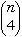Quandaries and Queries David Tricky logic/math question Parent Say you have a cirlcle. Then you draw 2 dots on the circle. Then you connect the dots with lines. The circle is divided into 2 parts. If you do the same with 3 dots and connect each dot to each dot with a line then you get a circle with 4 parts. 4 dots with lines connecting all (6 lines) = 8 parts 5 dots with lines connecting all (10 lines) = 16 parts Ok so far, there seems to be a good pattern forming here 2=2, 3=4, 4=8, 5=16 Now it gets tricky:: 6 dots all connected by lines (15 lines) = NOT 32, Correct answer is 31 spaces 7 dots all connected by lines (21 lines) = 57 spaces >> STRANGE!! I can't figure out the pattern. I don't know higher math well enough to do calculus or anything like that. Is there a formula you can think of to predict how many spaces would be formed by 8 dots/(28 lines) ?? Thank you for your time. David Hi David, When you say "6 dots all connected by lines (15 lines) = NOT 32, Correct answer is 31 spaces", it could even be lower. If you place your 6 dots as the vertices of a regular hexagon, then the little triangle in the middle vanishes into a single point, and you are left with 30 parts. So actually, you want a formula for the maximum number of parts into which the circle can be subdivided by joining n points on its circumference. For this you use Euler's formula: F + V = E + 2, where V is the number of "vertices", that is, your original n dots and the new points obtained as intersections of lines E is the number of "edges", that is, the lines joining two points. F is the number of "faces", that is, the parts of the circle and the outside face. What you really want is F - 1 (because you don't count the outside face), and the above formula can be rewritten as F - 1 = E - V + 1. Of this, V is the easiest to count: you start with n points, and every time you have 4 points on the circle, then you get a point of intersection of the two lines joining opposite pairs of points. There are= n(n-1)(n-2)(n-3)/4*3*2*1 ways to select 4 points amongst your n points on the circle, so you get V = n + n(n-1)(n-2)(n-3)/4*3*2*1. Counting the edges is a bit more tricky. It is best to fix a vertex and count its "degree", that is, the number of edges incident to it. There are two cases: If your vertex is one of the "new" vertices inside the circle, then there are lines starting from it and going towards 4 points on the circle, so its degree is 4. If your vertex is one of the n "original" dots, then there are lines starting from it and going to the other n-1 points, plus two arcs of circles connecting it to the two neighbouring dots. Adding these up, you get a degree of n+1. In all, you get n(n-1)(n-2)(n-3)/4*3*2*1 "new vertices of degree 4 and n vertices of degree n+1, so when you add up all the degrees you get [n(n-1)(n-2)(n-3)/4*3*2*1]*4 + n*(n+1). This is not the number of edges, but rather twice the number of edges, because each edge contributed two units to this sum: it got counted once in the degree of one of its end vertex, and once again in the degree of its other end vertex. Therefore 2*E = [n(n-1)(n-2)(n-3)/4*3*2*1]*4 + n*(n+1); dividing both sides by 2 we get E = [n(n-1)(n-2)(n-3)/4*3*2*1]*2 + n*(n+1)/2. Now that we have formulaes for V and E, we can substitute them into the formula F - 1 = E - V + 1; we get F - 1 = [n(n-1)(n-2)(n-3)/4*3*2*1]*2 + n*(n+1)/2 - [n + n(n-1)(n-2)(n-3)/4*3*2*1] + 1. It would be a bit strenuous to use this expression directly to find F - 1 once we have n, but we can simplify the expression by expanding and cancelling terms. I spare you the details, but the end result is F - 1 = (n4 -6n3 +23n2 -18n + 24)/24. You can check that this gives you the right number of parts for n from 2 to 7, including 31 for 6 and 57 for 7. Furthermore, it gives you 99 for 8, 163 for 9 and 256 for 10. It would be very difficult to make a drawing and count the parts. Claude Go to Math Central